Share

# HC Verma solutions for Class 12 Concepts of Physics Vol. 2 chapter 12 - Magnetic Field [Latest edition]

Textbook page

#### Chapters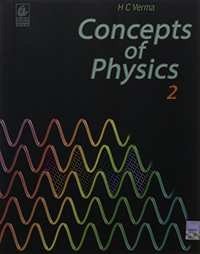## Chapter 12: Magnetic Field

### HC Verma solutions for Class 12 Concepts of Physics Vol. 2 Chapter 12 Magnetic Field Short Answers [Pages 228 - 229]

Short Answers | Q 1 | Page 228

Suppose a charged particle moves with a velocity v near a wire carrying an electric current. So, a magnetic force acts on it. If the same particle is seen from a frame moving with velocity v in the same direction, the charge will be found to be at rest. Will the magnetic force become zero in this frame?  Will the magnetic field become zero in this frame?

Short Answers | Q 2 | Page 229

Can a charged particle be accelerated by a magnetic field? Can its speed be increased?

Short Answers | Q 3 | Page 229

Will a current loop placed in a magnetic field always experience a zero force?

Short Answers | Q 4 | Page 229

The free electrons in a conducting wire are in constant thermal motion. If such a wire, carrying no current, is placed in a magnetic field, is there a magnetic force on each free electron? Is there a magnetic force on the wire?

Short Answers | Q 5 | Page 229

Assume that the magnetic field is uniform in a cubical region and zero outside. Can you project a charged particle from outside into the field, so that the particle describes a complete circle in the field?

Short Answers | Q 6 | Page 229

An electron beam projected along the positive x-axis deflects along the positive y-axis. If this deflection is caused by a magnetic field, what is the direction of the field? Can we conclude that the field is parallel to the z-axis?

Short Answers | Q 7 | Page 229

Is it possible for a current loop to stay without rotating in a uniform magnetic field? If yes, what should be the orientation of the loop?

Short Answers | Q 8 | Page 229

The net charge in a current-carrying wire is zero. Then, why does a magnetic field exert a force on it?

Short Answers | Q 9 | Page 229

The torque on a current loop is zero if the angle between the positive normal and the magnetic field is either θ = 0 or θ = 180°. In which of the two orientations, the equilibrium is stable?

Short Answers | Q 10 | Page 229

Verify that the units weber and volt second are the same.

### HC Verma solutions for Class 12 Concepts of Physics Vol. 2 Chapter 12 Magnetic Field MCQ [Pages 0 - 229]

MCQ | Q 1 | Page 229

A positively-charged particle projected towards east is deflected towards north by a magnetic field. The field may be

• towards west

• towards south

• upwards

• downwards

MCQ | Q 2 | Page 229

A charged particle is whirled in a horizontal circle on a frictionless table by attaching it to a string fixed at one point. If a magnetic field is switched on in the vertical direction, the tension in the string

• will increase

• will decrease

• will remain the same

• may increase or decrease

MCQ | Q 3 | Page 229

Which of the following particles will experience maximum magnetic force (magnitude) when projected with the same velocity perpendicular to a magnetic field?

• Electron

• Proton

• He+

•  Li++

MCQ | Q 4 | Page 229

Which of the following particles will describe the smallest circle when projected with the same velocity perpendicular to a magnetic field?

• Electron

•  Proton

• He+

•  Li+

MCQ | Q 5 | Page 229

Which of the following particles will have minimum frequency of revolution when projected with the same velocity perpendicular to a magnetic field?

•  Electron

• Proton

• He+

• Li+

MCQ | Q 6 | Page 229

A circular loop of area 1 cm2, carrying a current of 10 A, is placed in a magnetic field of 0.1 T perpendicular to the plane of the loop. The torque on the loop due to the magnetic field is

• zero

• 10−4 N m

• 10−2 N m

• 1 N m

MCQ | Q 7 | Page 229

A beam consisting of protons and electrons moving at the same speed goes through a thin region in which there is a magnetic field perpendicular to the beam. The protons and the electrons

• will go undeviated

• will be deviated by the same angle and will not separate

• will be deviated by different angles and hence will separate

•  will be deviated by the same angle but will separate

MCQ | Q 8

A charged particle moves in a uniform magnetic field. The velocity of the particle at some instant makes an acute angle with the magnetic field. The path of the particle will be

• a straight line

• a circle

• a helix with uniform pitch

•  a helix with non-uniform pitch

MCQ | Q 9 | Page 229

A particle moves in a region with a uniform magnetic field and a parallel, uniform electric field. At some instant, the velocity of the particle is perpendicular to the field direction. The path of the particle will be

• a straight line

•  a circle

• a helix with uniform pitch

•  a helix with non-uniform pitch

MCQ | Q 10 | Page 229

An electric current i enters and leaves a uniform circular wire of radius a through diametrically opposite points. A charged particle q, moving along the axis of the circular wire, passes through its centre at speed v. The magnetic force acting on the particle, when it passes through the centre, has a magnitude equal to

• qv(mu_0i)/(2a)

• qv(mu_0i)/(2pia)

• qv(mu_0i)/(a)

• Zero

### HC Verma solutions for Class 12 Concepts of Physics Vol. 2 Chapter 12 Magnetic Field MCQ [Pages 229 - 230]

MCQ | Q 1 | Page 229

If a charged particle at rest experiences no electromagnetic force,
(a) the electric field must be zero
(b) the magnetic field must be zero
(c) the electric field may or may not be zero
(d) the magnetic field may or may not be zero

MCQ | Q 2 | Page 230

If a charged particle kept at rest experiences an electromagnetic force,
(a) there must be an electric field
(b) there must be a magnetic field
(c) both fields cannot be zero
(d) both fields can be non-zero

MCQ | Q 3 | Page 230

If a charged particle projected in a gravity-free room deflects,
(a) there must be an electric field
(b) there must be a magnetic field
(c) both fields cannot be zero
(d) both fields can be non-zero

MCQ | Q 4 | Page 230

A charged particle moves in a gravity-free space without change in velocity. Which of the following is/are possible?
(a) E = 0, B = 0
(b) E = 0, B ≠ 0
(c) E ≠ 0, B = 0
(d) E ≠ 0, B ≠ 0

MCQ | Q 5 | Page 230

A charged particle moves along a circle under the action of possible constant electric and magnetic fields. Which of the following is possible?
(a) E = 0, B = 0
(b) E = 0, B ≠ 0
(c) E ≠ 0, B = 0
(d) E ≠ 0, B ≠ 0

MCQ | Q 6 | Page 230

A charged particle goes undeflected in a region containing an electric and a magnetic field. It is possible that
(a) vecE" || "vecB , vecv" || " vec E
(b) vecE  "is not parallel"  vecB
(c) vecv " || " vecB  but  vecv  "is not parallel"
(d) vecE" || " vecB  but   vecv "is not parallel"

MCQ | Q 7 | Page 230

If a charged particle moves unaccelerated in a region containing electric and magnetic fields
(a) vecE  "must be perpendicular" to vecB
(b) vecv "must be perpendicular" to vecE
(c) must be perpendicular to v_B

MCQ | Q 8 | Page 230

Two ions have equal masses but one is singly-ionised and the other is doubly-ionised. They are projected from the same place in a uniform magnetic field with the same velocity perpendicular to the field.
(a) Both ions will move along circles of equal radii.
(b) The circle described by the singly-ionised charge will have a radius that is double that of the other circle.
(c) The two circles do not touch each other.
(d) The two circles touch each other.

MCQ | Q 9 | Page 230

An electron is moving along the positive x-axis. You want to apply a magnetic field for a short time so that the electron may reverse its direction and move parallel to the negative x-axis. This can be done by applying the magnetic field along
(a) y-axis
(b) z-axis
(c) y-axis only
(d) z-axis only

MCQ | Q 10 | Page 230

and vecE and  vecBdenote electric and magnetic fields in a frame S and vecE→ and vecB in another frame S' moving with respect to S at a velocity vecV Two of the following equations are wrong. Identify them.
(a) B_y^, =  B_y + (vE_z)/c^2

(b) E_y^' = E_y - (vB_z)/(c^2)

(c) Ey = By + vE_z

(d) E_y = E_y + vB_z

### HC Verma solutions for Class 12 Concepts of Physics Vol. 2 Chapter 12 Magnetic Field [Pages 230 - 234]

Q 1 | Page 230

An alpha particle is projected vertically upward with a speed of 3.0 × 104 km s−1 in a region where a magnetic field of magnitude 1.0 T exists in the direction south to north. Find the magnetic force that acts on the α-particle.

Q 2 | Page 230

An electron is projected horizontally with a kinetic energy of 10 keV. A magnetic field of strength 1.0 × 10−7 T exists in the vertically upward direction. (a) Will the electron deflect towards the right or left of its motion? (b) Calculate the sideways deflection of the electron while travelling through 1 m. Make appropriate approximations.

Q 3 | Page 230

A magnetic field of $(4.0\times10^-3 \overrightarrow k)$ T exerts a force of $(4.0 \overrightarrow i + 3.0 \overrightarrow j ) \times 10^{−10} N$ on a particle with a charge of 1.0 × 10−9 C and going in the x − y plane. Find the velocity of the particle.

Q 4 | Page 230

An experimenter's diary reads as follows: "A charged particle is projected in a magnetic field of (7.0 vec i - 3.0 vecj)xx 10^-3 T. The acceleration of the particle is found to be (x veci + 7.0 vecj ) The number to the left of i in the last expression was not readable. What can this number be?

Q 5 | Page 230

A 10 g bullet with a charge of 4.00 μC is fired at a speed of 270 m s−1 in a horizontal direction. A vertical magnetic field of 500 µT exists in the space. Find the deflection of the bullet due to the magnetic field as it travels through 100 m. Make appropriate approximations.

Q 6 | Page 230

When a proton is released from rest in a room, it starts with an initial acceleration a0towards west. When it is projected towards north with a speed v0, it moves with an initial acceleration 3a0 towards west. Find the electric field and the maximum possible magnetic field in the room.

Q 7 | Page 230

Consider a 10-cm long portion of a straight wire carrying a current of 10 A placed in a magnetic field of 0.1 T making an angle of 53° with the wire. What magnetic force does the wire experience?

Q 8 | Page 230

A current of 2 A enters at the corner d of a square frame abcd of side 20 cm and leaves at the opposite corner b. A magnetic field B = 0.1 T exists in the space in a direction perpendicular to the plane of the frame, as shown in the figure. Find the magnitude and direction of the magnetic forces on the four sides of the frame.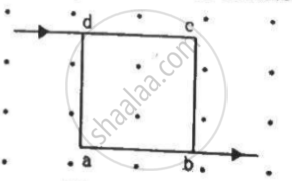Q 9 | Page 231

A magnetic field of strength 1.0 T is produced by a strong electromagnet in a cylindrical region of radius 4.0 cm, as shown in the figure. A wire, carrying a  current of 2.0 A, is placed perpendicular to and intersecting the axis of the cylindrical region. Find the magnitude of the force acting on the wire.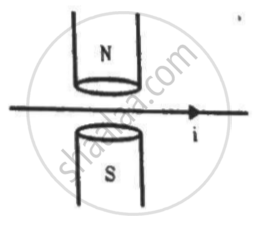Q 10 | Page 231

A wire of length l carries a current i long the x-axis. A magnetic field exists, which is given as vecB = B_0 (veci + vecj + veck)  T. Find the magnitude of the magnetic force acting on the wire.

Q 11 | Page 231

A current of 5.0 A exists in the circuit shown in the figure. The wire PQ has a length of 50 cm and the magnetic field in which it is immersed has a magnitude of 0.20 T. Find the magnetic force acting on the wire PQ.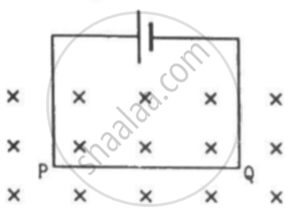Q 12 | Page 231

A circular loop of radius a, carrying a current i, is placed in a two-dimensional magnetic field. The centre of the loop coincides with the centre of the field (figure). The strength of the magnetic field at the periphery of the loop is B. Find the magnetic force on the wire.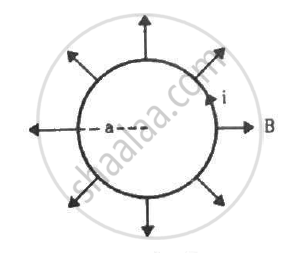Q 13 | Page 231

A hypothetical magnetic field existing in a region is given by vecB = B_0 vece where vece_r denotes the unit vector along the radial direction. A circular loop of radius a, carrying a current i, is placed with its plane parallel to the xy plane and the centre at (0, 0, d). Find the magnitude of the magnetic force acting on the loop.

Q 14 | Page 231

A rectangular wire-loop of width a is suspended from the insulated pan of a spring balance, as shown in the figure. A current exists in the anti-clockwise direction in the loop. A magnetic field B exists in the lower region. Find the change in the tension of the spring if the current in the loop is reversed.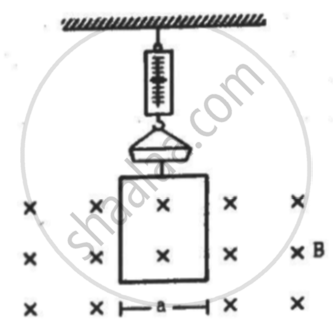Q 15 | Page 231

A current loop of arbitrary shape lies in a uniform magnetic field B. Show that the net magnetic force acting on the loop is zero.

Q 16 | Page 231

Prove that the force acting on a current-carrying wire, joining two fixed points a and b in a uniform magnetic field, is independent of the shape of the wire.

Q 17 | Page 231

A semicircular wire of radius 5.0 cm carries a current of 5.0 A. A magnetic field B of magnitude 0.50 T exists along the perpendicular to the plane of the wire. Find the magnitude of the magnetic force acting on the wire.

Q 18 | Page 231

A wire, carrying a current i, is kept in the xy plane along the curve y = A sin ((2x)/lamda x). magnetic field B exists in the z direction. Find the magnitude of the magnetic force on the portion of the wire between x = 0 and x = λ.

Q 19 | Page 231

A rigid wire consists of a semi-circular portion of radius R and two straight sections (figure). The wire is partially immersed in a perpendicular magnetic field B, as shown in the figure. Find the magnetic force on the wire if it carries a current i.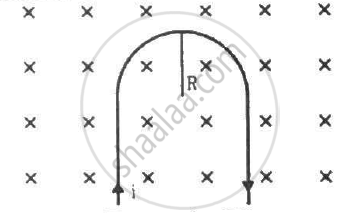Q 20 | Page 231

A straight horizontal wire of mass 10 mg and length 1.0 m carries a current of 2.0 A. What minimum magnetic field B should be applied in the region, so that the magnetic force on the wire may balance its weight?

Q 21 | Page 231

Figure shows a rod PQ of length 20.0 cm and mass 200 g suspended through a fixed point O by two threads of lengths 20.0 cm each. A magnetic field of strength 0.500 T exists in the vicinity of the wire PQ, as shown in the figure. The wires connecting PQ with the battery are loose and exert no force on PQ. (a) Find the tension in the threads when the switch is open. (b) A current of 2.0 A is established when the switch S is closed. Find the tension in the threads now.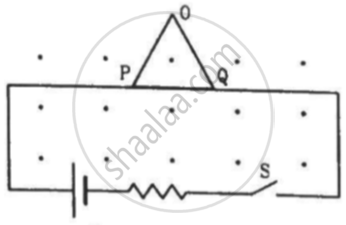Q 22 | Page 232

Two metal strips, each of length l, are clamped parallel to each other on a horizontal floor with a separation b between them. A wire of mass m lies on them perpendicularly, as shown in the figure. A vertically-upward magnetic field of strength B exists in the space. The metal strips are smooth but the coefficient of friction between the wire and the floor is µ. A current i is established when the switch S is closed at the instant t = 0. Discuss the motion of the wire after the switch is closed. How far away from the strips will the wire reach?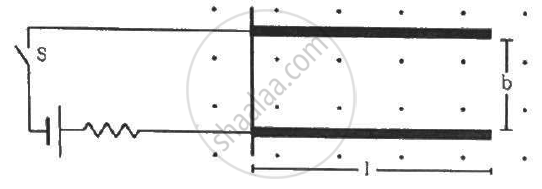Q 23 | Page 232

A metal wire PQ of mass 10 g lies at rest on two horizontal metal rails separated by 4.90 cm (figure). A vertically-downward magnetic field of magnitude 0.800 T exists in the space. The resistance of the circuit is slowly decreased and it is found that when the resistance goes below 20.0 Ω, the wire PQ starts sliding on the rails. Find the coefficient of friction.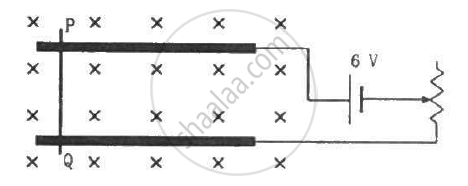Q 24 | Page 232

A straight wire of length l can slide on two parallel plastic rails kept in a horizontal plane with a separation d. The coefficient of friction between the wire and the rails is µ. If the wire carries a current i, what minimum magnetic field should exist in the space in order to slide the wire on the rails?

Q 25 | Page 232

The figure shows a circular wire loop of radius a and carrying a current i, which is placed in a perpendicular magnetic field B. (a) Consider a small part dl of the wire. Find the force on this part of the wire exerted by the magnetic field. (b) Find the force of compression in the wire.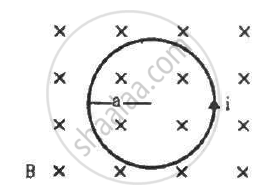Q 26 | Page 232

Suppose that the radius of cross-section of the wire used in the previous problem is r. Find the increase in the radius of the loop if the magnetic field is switched off. Young's modulus of the material of the wire is Y.

Q 27 | Page 232

The magnetic field existing in a region is given by  vecB = B_0(1 + x/1)veck . A square loop of edge l and carrying a current i, is placed with its edges parallel to the xy axes. Find the magnitude of the net magnetic force experienced by the loop.

Q 28 | Page 232

A conducting wire of length l, lying normal to a magnetic field B, moves with a velocity v,as shown in the figure. (a) Find the average magnetic force on a free electron of the wire. (b) Due to this magnetic force, electrons concentrate at one end, resulting in an electric field inside the wire. The redistribution stops when the electric force on the free electrons balances the magnetic force. Find the electric field developed inside the wire when the redistribution stops. (c) What potential difference is developed between the ends of the wire?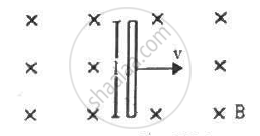Q 29 | Page 232

A current i is passed through a silver strip of width d and area of cross-section A. The number of free electrons per unit volume is n. (a) Find the drift velocity v of the electrons. (b) If a magnetic field B exists in the region, as shown in the figure, what is the average magnetic force on the free electrons? (c) Due to the magnetic force, the free electrons get accumulated on one side of the conductor along its length. This produces a transverse electric field in the conductor, which opposes the magnetic force on the electrons. Find the magnitude of the electric field which will stop further accumulation of electrons. (d) What will be the potential difference developed across the width of the conductor due to the electron-accumulation? The appearance of a transverse emf, when a current-carrying wire is placed in a magnetic field, is called Hall effect.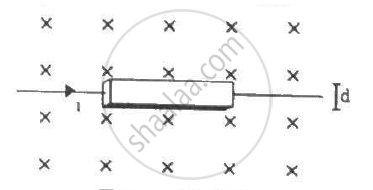Q 30 | Page 232

A particle of charge 2.0 × 10−8 C and mass 2.0 × 10−10 g is projected with a speed of 2.0 × 103 m s−1 in a region with a uniform magnetic field of 0.10 T. The velocity is perpendicular to the field. Find the radius of the circle formed by the particle and also the time period.

Q 31 | Page 232

A proton describes a circle of radius 1 cm in a magnetic field of strength 0.10 T. What would be the radius of the circle described by an α-particle moving with the same speed in the same magnetic field?

Q 32 | Page 232

An electron of kinetic energy 100 eV circulates in a path of radius 10 cm in a magnetic field. Find the magnetic field and the number of revolutions per second made by the electron.

Q 33 | Page 232

Protons with kinetic energy K emerge from an accelerator as a narrow beam. The beam is bent by a perpendicular magnetic field, so that it just misses a plane target kept at a distance l in front of the accelerator. Find the magnetic field.

Q 34 | Page 233

A charged particle is accelerated through a potential difference of 12 kV and acquires a speed of 1.0 × 106 m s−1. It is then injected perpendicularly into a magnetic field of strength 0.2 T. Find the radius of the circle described by it.

Q 35 | Page 233

Doubly-ionised helium ions are projected with a speed of 10 km s−1 in a direction perpendicular to a uniform magnetic field of magnitude 1.0 T. Find (a) the force acting on an ion (b) the radius of the circle in which it circulates and (c) the time taken by an ion to complete the circle.

Q 36 | Page 233

A proton is projected with a velocity of 3 × 106 m s−1 perpendicular to a uniform magnetic field of 0.6 T. Find the acceleration of the proton.

Q 37 | Page 233

(a) An electron moves along a circle of radius 1 m in a perpendicular magnetic field of strength 0.50 T. What would be its speed? Is it reasonable? (b) If a proton moves along a circle of the same radius in the same magnetic field, what would be its speed?

Q 38 | Page 233

A particle of mass m and positive charge q, moving with a uniform velocity v, enters a magnetic field B, as shown in the figure. (a) Find the radius of the circular arc it describes in the magnetic field. (b) Find the angle subtended by the arc at the centre. (c) How long does the particle stay inside the magnetic field? (d) Solve the three parts of the above problem if the charge q on the particle is negative.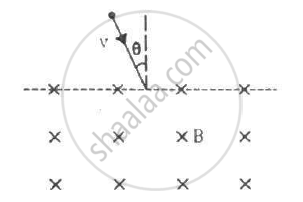Q 39 | Page 233

A particle of mass m and charge q is projected into a region that has a perpendicular magnetic field B. Find the angle of deviation (figure) of the particle as it comes out of the magnetic field if the width d of the region is very slightly smaller than

(a) (mv)/(qB)  (b)(mv)/(2qB) (c)(2mv)/(qB)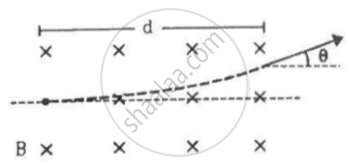Q 40 | Page 233

A narrow beam of singly-charged carbon ions, moving at a constant velocity of 6.0 × 104m s−1, is sent perpendicularly in a rectangular region of uniform magnetic field B = 0.5 T (figure). It is found that two beams emerge from the field in the backward direction, the separations from the incident beam being 3.0 cm and 3.5 cm. Identify the isotopes present in the ion beam. Take the mass of an ion = A(1.6 × 10−27) kg, where A is the mass number.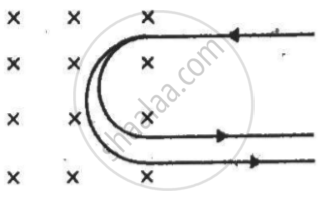Q 41 | Page 233

Fe+ ions are accelerated through a potential difference of 500 V and are injected normally into a homogeneous magnetic field B of strength 20.0 mT. Find the radius of the circular paths followed by the isotopes with mass numbers 57 and 58. Take the mass of an ion = A (1.6 × 10−27) kg, where A is the mass number.

Q 42 | Page 233

A narrow beam of singly charged potassium ions of kinetic energy 32 keV is injected into a region of width 1.00 cm with a magnetic field of strength 0.500 T, as shown in the figure. The ions are collected at a screen 95.5 cm away from the field region. If the beam contains isotopes of atomic weights 39 and 41, find the separation between the points where these isotopes strike the screen. Take the mass of a potassium ion = A (1.6 × 10−27) kg, where A is the mass number.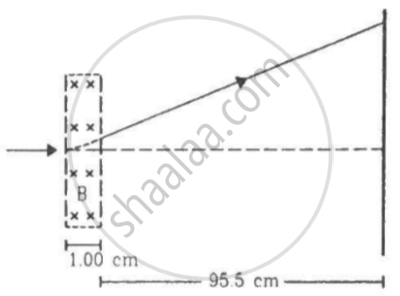Q 43 | Page 233

The figure shows a convex lens of focal length 12 cm lying in a uniform magnetic field Bof magnitude 1.2 T parallel to its principal axis. A particle with charge 2.0 × 10−3 C  and mass 2.0 × 10−5 kg is projected perpendicular to the plane of the diagram with a speed of 4.8 m s−1. The particle moves along a circle with its centre on the principal axis at a distance of 18 cm from the lens. Show that the image of the particle moves along a circle and find the radius of that circle.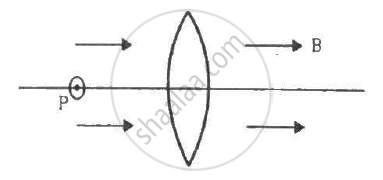Q 44 | Page 233

Electrons emitted with negligible speed from an electron gun are accelerated through a potential difference V along the x-axis. These electrons emerge from a narrow hole into a uniform magnetic field B directed along this axis. However, some of the electrons emerging from the hole make slightly divergent angles, as shown in the figure. Show that these paraxial electrons are refocussed on the x-axis at a distance sqrt(8pi^2mV)/(eB^2).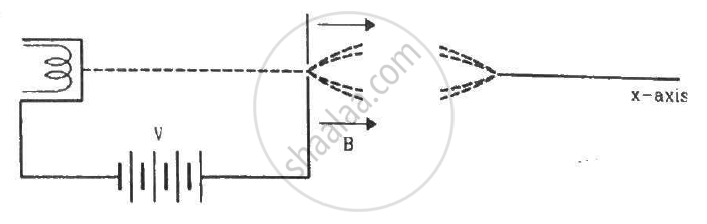Q 45 | Page 234

Two particles, each with mass m are placed at a separation d in a uniform magnetic field B, as shown in the figure. They have opposite charges of equal magnitude q. At time t = 0, the particles are projected towards each other, each with a speed v. Suppose the Coulomb force between the charges is switched off. (a) Find the maximum value vmof the projection speed, so that the two particles do not collide. (b) What would be the minimum and maximum separation between the particles if v = vm/2? (c) At what instant will a collision occur between the particles if v = 2vm? (d) Suppose v = 2vm and the collision between the particles is completely inelastic. Describe the motion after the collision.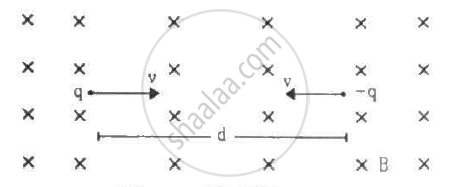Q 46 | Page 234

A uniform magnetic field of magnitude 0.20 T exists in space from east to west. With what speed should a particle of mass 0.010 g and with charge 1.0 × 10−5 C be projected from south to north so that it moves with uniform velocity?

Q 47 | Page 234

A particle moves in a circle of diameter 1.0 cm under the action of a magnetic field of 0.40 T. An electric field of 200 V m−1 makes the path straight. Find the charge/mass ratio of the particle.

Q 48 | Page 234

A proton goes undeflected in a crossed electric and magnetic field (the fields are perpendicular to each other) at a speed of 2.0 × 105 m s−1. The velocity is perpendicular to both the fields. When the electric field is switched off, the proton moves along a circle of radius 4.0 cm. Find the magnitudes of the electric and magnetic fields. Take the mass of the proton = 1.6 × 10−27 kg

Q 49 | Page 233

A particle with a charge of 5.0 µC and a mass of 5.0 × 10−12 kg is projected with a speed of 1.0 km s−1 in a magnetic field of magnitude 5.0 mT. The angle between the magnetic field and the velocity is sin−1 (0.90). Show that the path of the particle will be a helix. Find the diameter of the helix and its pitch.

Q 50 | Page 234

A proton projected in a magnetic field of 0.020 T travels along a helical path of radius 5.0 cm and pitch 20 cm. Find the components of the velocity of the proton along and perpendicular to the magnetic field. Take the mass of the proton = 1.6 × 10−27 kg

Q 51 | Page 234

A particle of mass m and charge q is released from the origin in a region in which the electric field and magnetic field are given by
vecB = -B_0 vecj and vecE = E_0 vecK
Find the speed of the particle as a function of its z-coordinate.

Q 52 | Page 234

An electron is emitted with negligible speed from the negative plate of a parallel-plate capacitor charged to a potential difference V. The separation between the plates is dand a magnetic field B exists in the space, as shown in the figure. Show that the electron will fail to strike the upper plates if d > ((2m_eV)/(eB_0^2))^(1/2)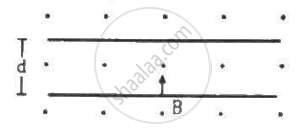Q 53 | Page 234

A rectangular coil of 100 turns has length 5 cm and width 4 cm. It is placed with its plane parallel to a uniform magnetic field and a current of 2 A is sent through the coil. Find the magnitude of the magnetic field B if the torque acting on the coil is 0.2 N m−1

Q 54 | Page 234

A 50-turn circular coil of radius 2.0 cm carrying a current of 5.0 A is rotated in a magnetic field of strength 0.20 T. (a) What is the maximum torque that acts on the coil? (b) In a particular position of the coil, the torque acting on it is half of this maximum. What is the angle between the magnetic field and the plane of the coil?

Q 55 | Page 234

A rectangular loop of sides 20 cm and 10 cm carries a current of 5.0 A. A uniform magnetic field of magnitude  0.20 T exists parallel to the longer side of the loop. (a) What is the force acting on the loop? (b) What is the torque acting on the loop?

Q 56 | Page 234

A circular coil of radius 2.0 cm has 500 turns and carries a current of 1.0 A. Its axis makes an angle of 30° with the uniform magnetic field of magnitude 0.40 T that exists in the space. Find the torque acting on the coil.

Q 57 | Page 234

A circular loop carrying a current i is made of a wire of length L. A uniform magnetic field B exists parallel to the plane of the loop. (a) Find the torque on the loop. (b) If the same length of the wire is used to form a square loop, what would be the torque? Which is larger?

Q 58 | Page 234

A square coil of edge l and with n turns carries a current i. It is kept on a smooth horizontal plate. A uniform magnetic field B exists parallel to an edge. The total mass of the coil is M. What should be the minimum value of B for which the coil will start tipping over?

Q 59 | Page 234

Consider a non-conducting ring of radius r and mass m that has a total charge qdistributed uniformly on it. The ring is rotated about its axis with an angular speed ω. (a) Find the equivalent electric current in the ring. (b) Find the magnetic moment µ of the ring. (c) Show that pi = (q)/(2m) l, where l is the angular momentum of the ring about its axis of rotation.

Q 60 | Page 234

Consider a non-conducting plate of radius r and mass m that has a charge q distributed uniformly over it. The plate is rotated about its axis with an angular speed ω. Show that the magnetic moment µ and the angular momentum l of the plate are related as mu = q/(2 m)l

Q 61 | Page 234

Consider a solid sphere of radius r and mass m that has a charge q distributed uniformly over its volume. The sphere is rotated about its diameter with an angular speed ω. Show that the magnetic moment µ and the angular momentum l of the sphere are related as mu = q/(2m) l

## Chapter 12: Magnetic Field## HC Verma solutions for Class 12 Concepts of Physics Vol. 2 chapter 12 - Magnetic Field

HC Verma solutions for Class 12 Concepts of Physics Vol. 2 chapter 12 (Magnetic Field) include all questions with solution and detail explanation. This will clear students doubts about any question and improve application skills while preparing for board exams. The detailed, step-by-step solutions will help you understand the concepts better and clear your confusions, if any. Shaalaa.com has the CBSE Class 12 Concepts of Physics Vol. 2 solutions in a manner that help students grasp basic concepts better and faster.

Further, we at Shaalaa.com provide such solutions so that students can prepare for written exams. HC Verma textbook solutions can be a core help for self-study and acts as a perfect self-help guidance for students.

Concepts covered in Class 12 Concepts of Physics Vol. 2 chapter 12 Magnetic Field are The Magnetic Dipole Moment of a Revolving Electron, Circular Current Loop as a Magnetic Dipole, Torque on a Rectangular Current Loop in a Uniform Magnetic Field, Magnetic Field on the Axis of a Circular Current Loop, Motion in a Magnetic Field, Velocity Selector, Solenoid and the Toroid - the Toroid, Solenoid and the Toroid - the Solenoid, Magnetic Diapole, Moving Coil Galvanometer, Torque on a Current Loop in Magnetic Field, Force Between Two Parallel Currents, the Ampere, Force on a Current - Carrying Conductor in a Uniform Magnetic Field, Cyclotron, Force on a Moving Charge in Uniform Magnetic and Electric Fields, Straight and Toroidal Solenoids (Only Qualitative Treatment), Ampere’s Circuital Law, Magnetic Field Due to a Current Element, Biot-Savart Law, Oersted’s Experiment, Magnetic Force.

Using HC Verma Class 12 solutions Magnetic Field exercise by students are an easy way to prepare for the exams, as they involve solutions arranged chapter-wise also page wise. The questions involved in HC Verma Solutions are important questions that can be asked in the final exam. Maximum students of CBSE Class 12 prefer HC Verma Textbook Solutions to score more in exam.

Get the free view of chapter 12 Magnetic Field Class 12 extra questions for Class 12 Concepts of Physics Vol. 2 and can use Shaalaa.com to keep it handy for your exam preparation